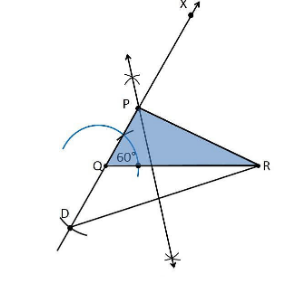Guru

# Construct a triangle PQR in which QR = 6cm, ∠Q = 60° and PR–PQ = 2cm. Q.3

• 0

Give me the best way for solving the question of class 9th of Constructions of exercise 11.2  of math. Give me the easiest way for solving this question in simple way of question no.3 Construct a triangle PQR in which QR = 6cm, ∠Q = 60° and PR–PQ = 2cm

Share

1. Construction Procedure:

The steps to draw the triangle of given measurement is as follows:

1. Draw a line segment of base QR = 6 cm

2. Measure and draw ∠Q = 60° and let the ray be QX

3. Take a compass and measure PR–PQ = 2cm.

4. Since PR–PQ is negative, QD will below the line QR.

5. With Q as centre and draw an arc at the point be D on the ray QX

6. Join DR

7. Now draw the perpendicular bisector of the line DR and the intersection point is taken as P.

8. Now join PR

9. Therefore, PQR is the required triangle.• 0